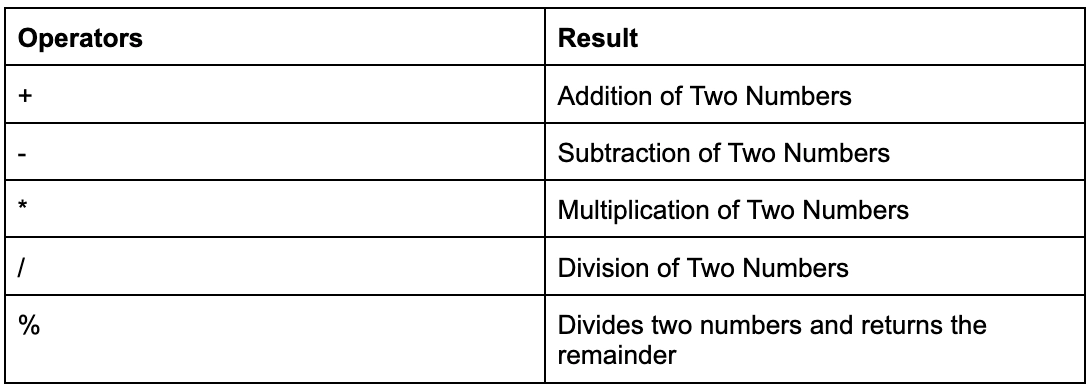New update is available. Click here to update.
Last Updated: Jul 4, 2023

# Arithmetic Operator0 upvote

## Introduction

A programmer often creates a program to execute some function. For example, we may simply add two integers in a program using the + symbol.
In this case, + is a symbol that performs an operation known as an addition. In Java, such symbols are referred to as operators.
An expression in Java consists of two parts: operand and operator. Operands are the variables or constants that operators act on.
In Java, an operator is a symbol or term instructing the compiler to do certain mathematical or logical operations.

Also see, Duck Number in Java and Hashcode Method in Java.

## Arithmetic Operators in Java

These operators are mathematical operators that may be used to execute simple or complicated arithmetic operations on the operands, which are primitive data types. These operators are a collection of unary and binary operators that may be used on one or two operands. Let's have a look at the different arithmetic operators that Java has to offer.This is a binary operator that adds two operands.

Syntax:

``num1 + num2``

Example:

``````import java.io.*;
public static void main(String[] args)
{
int num1 = 100, num2 = 200, sum = 0;
// Displaying the two numbers
System.out.println("num1 = " + num1);
System.out.println("num2 = " + num2);
sum = num1 + num2;
System.out.println("The sum = " + sum);
}
}``````

Output :

``````num1 = 100
num2 = 200
The sum = 300``````

### Subtraction(-)

This is a binary operator that subtracts two operands.

Syntax:

``num1 -  num2``

Example:

``````import java.io.*;
public class Subtraction{
public static void main(String[] args)
{
int num1 = 200, num2 = 100, diff = 0;

// Displaying the two numbers
System.out.println("num1 = " + num1);
System.out.println("num2 = " + num2);

// subtracting the two numbers
diff = num1 -  num2;
System.out.println("The Difference = " + diff);
}
}``````

Output :

``````num1 = 200
num2 = 100
The Difference = 100``````

You can also read about Java Destructor and Swap Function in Java

### Multiplication(*)

This is a binary operator that Multiplies two operands.

Syntax:

``num1 * num2``

Example:

``````import java.io.*;
public class Multiplication {
public static void main(String[] args)
{
int num1 = 60, num2 = 40, res = 0;
// Displaying the two numbers
System.out.println("num1 = " + num1);
System.out.println("num2 = " + num2);
// multiplying the two numbers
res= num1 * num2;
System.out.println("The Multuplication = " + res);
}
}``````

Output :

``````num1 = 60
num2 = 40
The Multiplication = 2400``````

### Division(/)

This is a binary operator that divides the first operand (dividend) by the second operand (divisor), resulting in the quotient.

Syntax:

``num1 / num2``

Example:

``````import java.io.*;
public class Division {
public static void main(String[] args)
{
int num1 = 200, num2 = 100, div = 0;
// Displaying the two numbers
System.out.println("num1 = " + num1);
System.out.println("num2 = " + num2);
// dividing the two numbers
div= num1 / num2;
System.out.println("The Division = " + div);
}
}``````

Output :

``````num1 = 200
num2 = 100
The Division = 2``````

### Modulus(%)

This is a binary operator that returns the remainder after dividing the first operand (dividend) by the second operand (divisor).

Syntax:

``num1 % num2``

Example:

``````import java.io.*;
public class Modulus{
public static void main(String[] args)
{
int num1 = 3, num2 = 2, mod = 0;
// Displaying the two numbers
System.out.println("num1 = " + num1);
System.out.println("num2 = " + num2);
// remaindering the two numbers
mod = num1 % num2;
System.out.println("The Remainder = " + mod);
}
}``````

Output :

``````num1 = 3
num2 = 2
The Remainder = 1``````

Compile it on online java editor.

Also check out Addition of Two Numbers in Java here.

1. What are the 4 operators in Java?
Java arithmetic operators are used to accomplish operations such as addition, subtraction, multiplication, and division.

2. What is the function of operators?
An operator is a tool that is used to change individual data elements and deliver a result. These things are referred to as operands or arguments. Special characters or keywords are used to represent operators.

3. What is an example of an operator?
An operator is defined as someone who controls a machine or the management or owner of a firm. A telephone switchboard operator is one example of an operator. An operator is someone who controls a crane at a loading dock, for example.

## Key Takeaways

In this article we have extensively discussed arithmatic operators topics and their implementation in Java.With the help of examples of each, we saw addition, subtraction, multiplication, division and modulus operators in detail.

Check out the java interview questions to get hands-on experience with frequently asked interview questions and land your dream job.